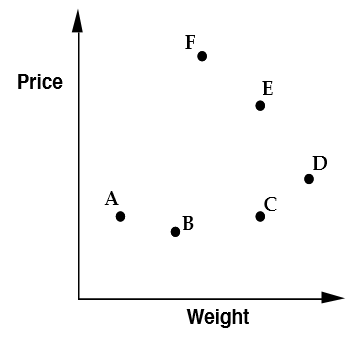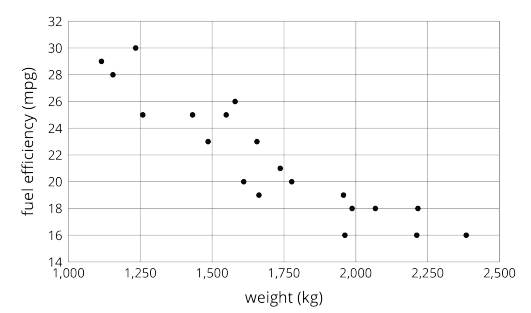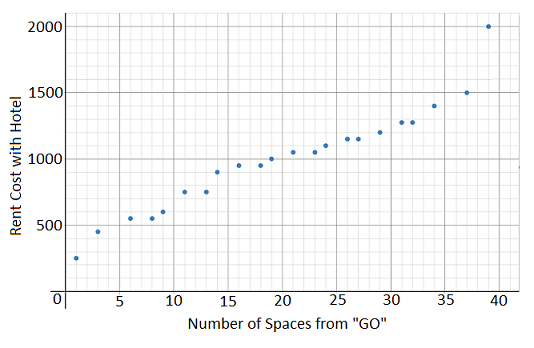# Bivariate Data

## Objective

Define bivariate data. Analyze data in scatter plots.

## Common Core Standards

### Core Standards

?

• 8.SP.A.1 — Construct and interpret scatter plots for bivariate measurement data to investigate patterns of association between two quantities. Describe patterns such as clustering, outliers, positive or negative association, linear association, and nonlinear association.

?

• 6.SP.B.4

## Criteria for Success

?

1. Understand scatter plots as a representation of bivariate data in two variables.
2. Understand that each ordered pair ${{(x, y)}}$ in a scatter plot represents one piece of the data set.
3. Interpret ordered pairs ${{(x, y)}}$ in scatter plots in context of the variables (MP.2).
4. Make initial observations of bivariate data represented in scatter plots.

## Tips for Teachers

?

• Lessons 1 and 2 introduce students to bivariate data as it is seen in scatter plots. Lesson 1 focuses on reading and interpreting scatter plots in order to analyze what information can be gleaned from them. In Lesson 2, students will construct scatter plots from data sets.
• Students are asked to describe and explain relationships they see in scatter plots. They have not discussed vocabulary such as linear, positive, etc., which will come in later lessons; for this lesson, allow students to use their own vocabulary to describe what they see.

#### Fishtank Plus

• Problem Set
• Student Handout Editor
• Vocabulary Package

## Anchor Problems

?

### Problem 1

Each point on this graph represents a bag of sugar.1. Which point shows the heaviest bag?
2. Which point shows the cheapest bag?
3. Which points show bags with the same weight?
4. Which points show bags with the same price?
5. Which of F or C gives the best value for the money? How can you tell?

#### References

MARS Summative Assessment Tasks for High School Sugar Prices

Sugar Prices from the Summative Tasks is made available through the Mathematics Assessment Project under the CC BY-NC-ND 3.0 license. Copyright © 2007-2015 Mathematics Assessment Resource Service, University of Nottingham. Accessed June 21, 2017, 10:59 a.m..

### Problem 2

The scatter plot shows weights and fuel efficiencies for 18 cars.1. What does the point ${(x, y)}$ represent in this context?

#### References

Open Up Resources Grade 8 Unit 6 Lesson 3Image from 3.2: Weight and Fuel Efficiency

## Problem Set

?The following resources include problems and activities aligned to the objective of the lesson that can be used to create your own problem set.

• Include examples where students are given scatter plots and asked questions similar to the Anchor Problems.

?

In the game of Monopoly, as you move around the board, the rental costs of properties with one hotel change. The scatter plot below shows some information about these rental costs compared to the number of spaces you are from the starting place, “GO.”1. What are the two variables in the scatter plot?
2. What is the cheapest rent for a Monopoly property with one hotel? What is the most expensive?
3. Are there any hotels that have the same rental price but are a different number of spaces from “GO”? If so, name the rent cost and number of spaces from “GO.”
4. Do you notice a relationship between the data? Explain.

?